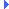FD.io VPP  v18.07.1-11-g31aa6f2 Vector Packet Processing
fib_walk.h File ReferenceInclude dependency graph for fib_walk.h:This graph shows which files directly or indirectly include this file:

Go to the source code of this file.

## Macros

#define FIB_WALK_PRIORITY_NUM   ((fib_walk_priority_t)(FIB_WALK_PRIORITY_LOW+1))

#define FIB_WALK_PRIORITIES

#define FOR_EACH_FIB_WALK_PRIORITY(_prio)

## Typedefs

typedef enum fib_walk_priority_t_ fib_walk_priority_t
Walk priorities. More...

## Enumerations

enum  fib_walk_priority_t_ { FIB_WALK_PRIORITY_HIGH = 0, FIB_WALK_PRIORITY_LOW = 1 }
Walk priorities. More...

## Functions

void fib_walk_module_init (void)

void fib_walk_async (fib_node_type_t parent_type, fib_node_index_t parent_index, fib_walk_priority_t prio, fib_node_back_walk_ctx_t *ctx)

void fib_walk_sync (fib_node_type_t parent_type, fib_node_index_t parent_index, fib_node_back_walk_ctx_t *ctx)
Back walk all the children of a FIB node. More...

u8format_fib_walk_priority (u8 *s, va_list *ap)

void fib_walk_process_enable (void)

void fib_walk_process_disable (void)

## Macro Definition Documentation

 #define FIB_WALK_PRIORITIES
Value:

Definition at line 34 of file fib_walk.h.

 #define FIB_WALK_PRIORITY_NUM   ((fib_walk_priority_t)(FIB_WALK_PRIORITY_LOW+1))

Definition at line 32 of file fib_walk.h.

 #define FOR_EACH_FIB_WALK_PRIORITY ( _prio )
Value:
for ((_prio) = FIB_WALK_PRIORITY_HIGH; \
(_prio) < FIB_WALK_PRIORITY_NUM; \
(_prio)++)
#define FIB_WALK_PRIORITY_NUM
Definition: fib_walk.h:32

Definition at line 39 of file fib_walk.h.

## Typedef Documentation

 typedef enum fib_walk_priority_t_ fib_walk_priority_t

Walk priorities.

Strict priorities. All walks a priority n are completed before n+1 is started. Increasing numerical value implies decreasing priority.

## Enumeration Type Documentation

 enum fib_walk_priority_t_

Walk priorities.

Strict priorities. All walks a priority n are completed before n+1 is started. Increasing numerical value implies decreasing priority.

Enumerator
FIB_WALK_PRIORITY_HIGH
FIB_WALK_PRIORITY_LOW

Definition at line 26 of file fib_walk.h.

## Function Documentation

 void fib_walk_async ( fib_node_type_t parent_type, fib_node_index_t parent_index, fib_walk_priority_t prio, fib_node_back_walk_ctx_t * ctx )

Definition at line 673 of file fib_walk.c.Here is the call graph for this function:Here is the caller graph for this function:

 void fib_walk_module_init ( void )

Definition at line 917 of file fib_walk.c.Here is the call graph for this function:Here is the caller graph for this function:

 void fib_walk_process_disable ( void )

Definition at line 1173 of file fib_walk.c.Here is the call graph for this function:Here is the caller graph for this function:

 void fib_walk_process_enable ( void )

Definition at line 1164 of file fib_walk.c.Here is the call graph for this function:Here is the caller graph for this function:

 void fib_walk_sync ( fib_node_type_t parent_type, fib_node_index_t parent_index, fib_node_back_walk_ctx_t * ctx )

Back walk all the children of a FIB node.

note this is a synchronous depth first walk. Children visited may propagate the walk to thier children. Other children node types may not propagate, synchronously but instead queue the walk for later async completion.

Definition at line 727 of file fib_walk.c.Here is the call graph for this function:Here is the caller graph for this function:

 u8* format_fib_walk_priority ( u8 * s, va_list * ap )

Definition at line 188 of file fib_walk.c.Here is the call graph for this function:Here is the caller graph for this function: